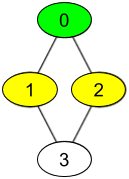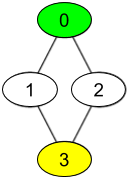# 获取你好友已观看的视频

## 1311. 获取你好友已观看的视频 (Medium)

Level 1 的视频包含所有你好友观看过的视频，level 2 的视频包含所有你好友的好友观看过的视频，以此类推。一般的，Level 为 k 的视频包含所有从你出发，最短距离为 k 的好友观看过的视频。```输入：watchedVideos = [["A","B"],["C"],["B","C"],["D"]], friends = [[1,2],[0,3],[0,3],[1,2]], id = 0, level = 1

id 为 1 -> watchedVideos = ["C"]
id 为 2 -> watchedVideos = ["B","C"]

B -> 1
C -> 2
``````输入：watchedVideos = [["A","B"],["C"],["B","C"],["D"]], friends = [[1,2],[0,3],[0,3],[1,2]], id = 0, level = 2

```

• `n == watchedVideos.length == friends.length`
• `2 <= n <= 100`
• `1 <= watchedVideos[i].length <= 100`
• `1 <= watchedVideos[i][j].length <= 8`
• `0 <= friends[i].length < n`
• `0 <= friends[i][j] < n`
• `0 <= id < n`
• `1 <= level < n`
• 如果 `friends[i]` 包含 `j` ，那么 `friends[j]` 包含 `i`

### 相关话题

[广度优先搜索] [哈希表] [字符串]